Check Full Chapter Explained - Continuity and Differentiability - Application of Derivatives (AOD) Class 12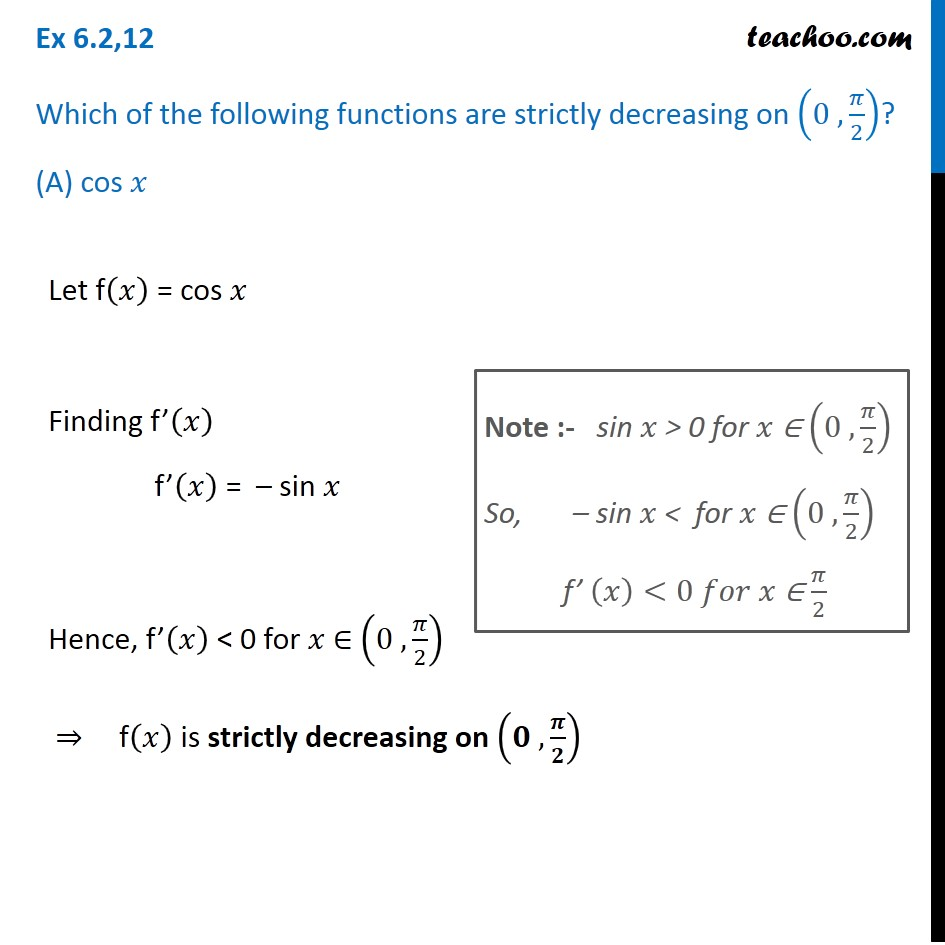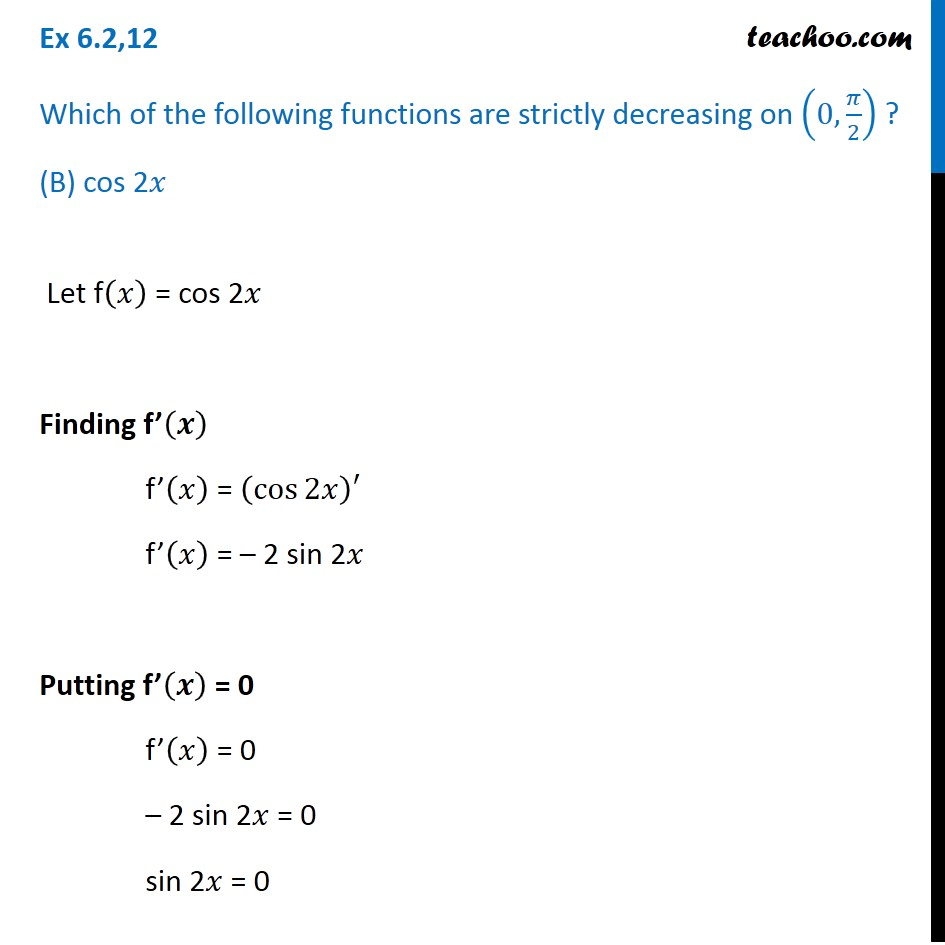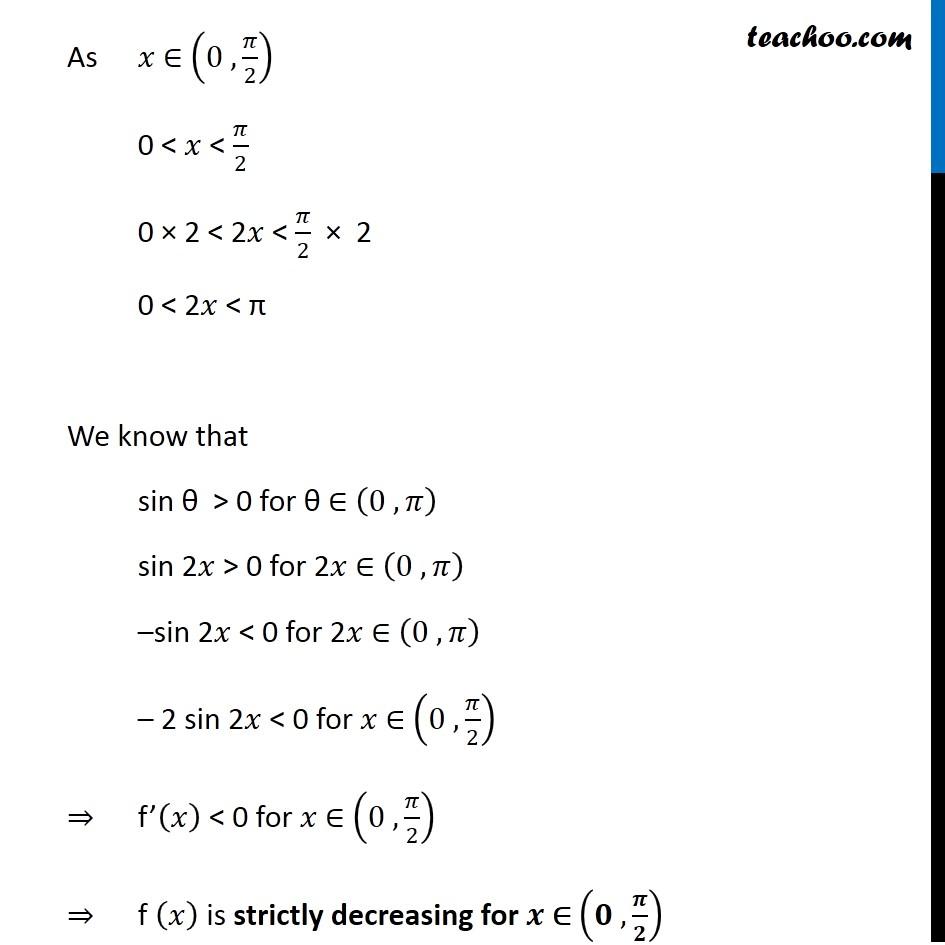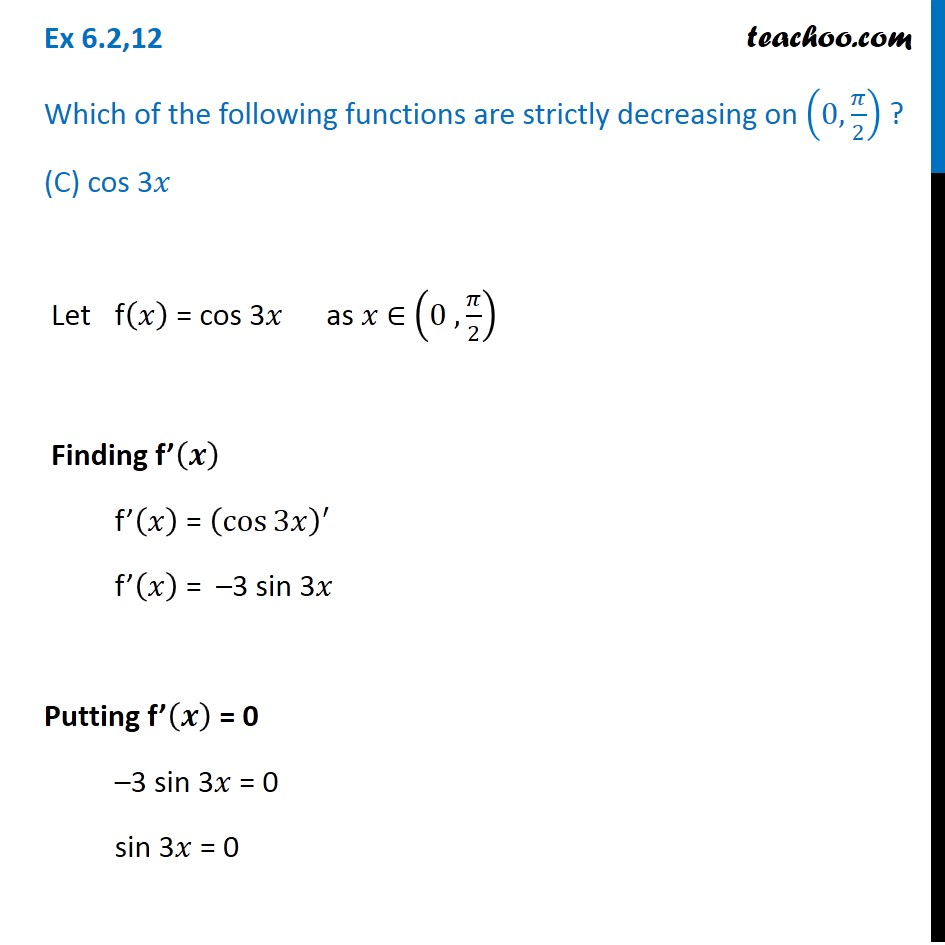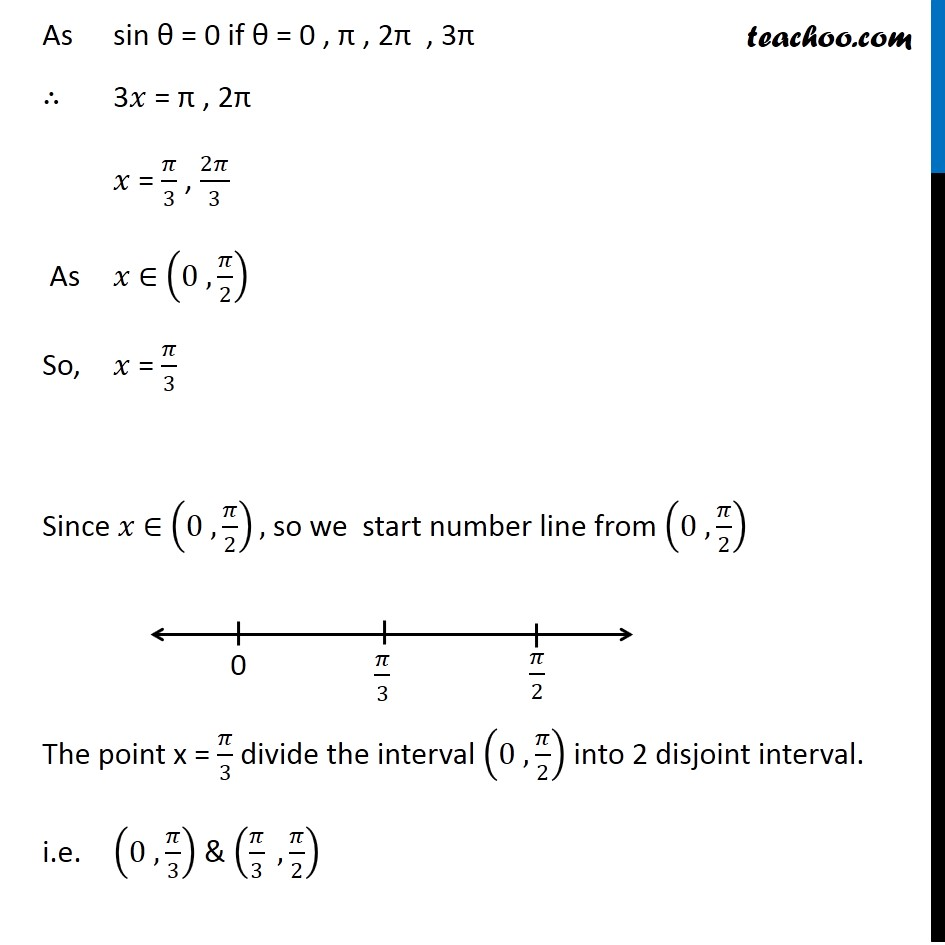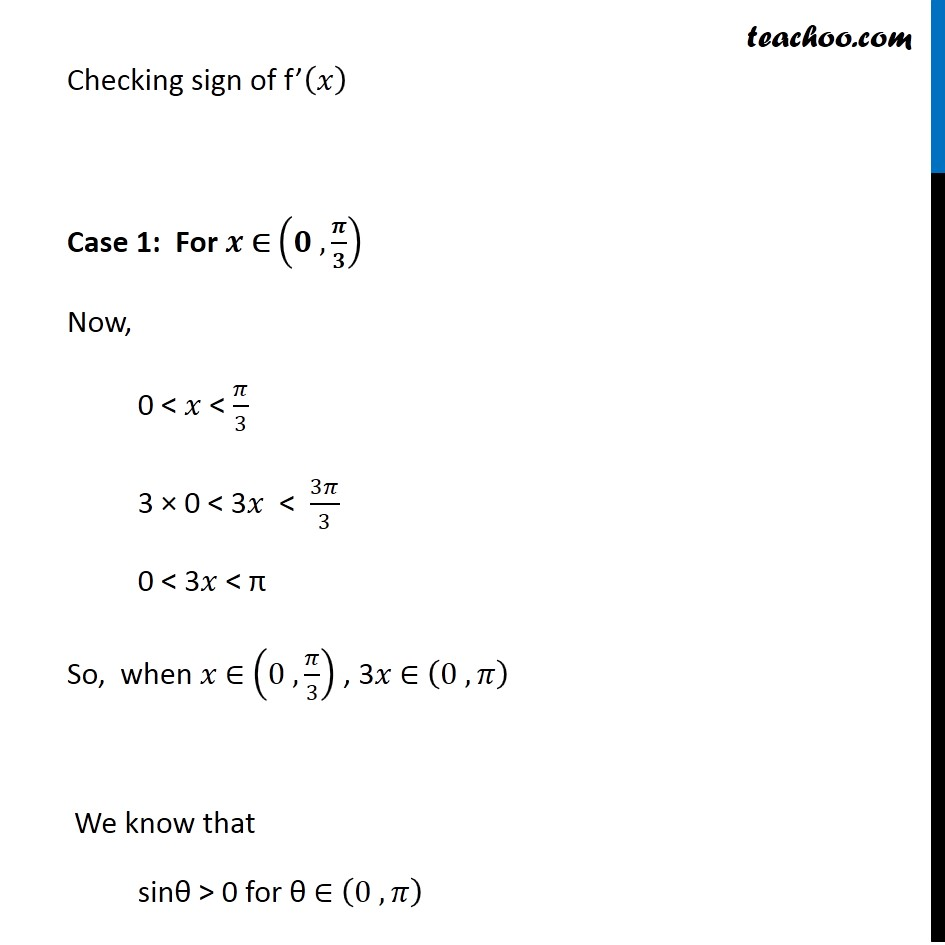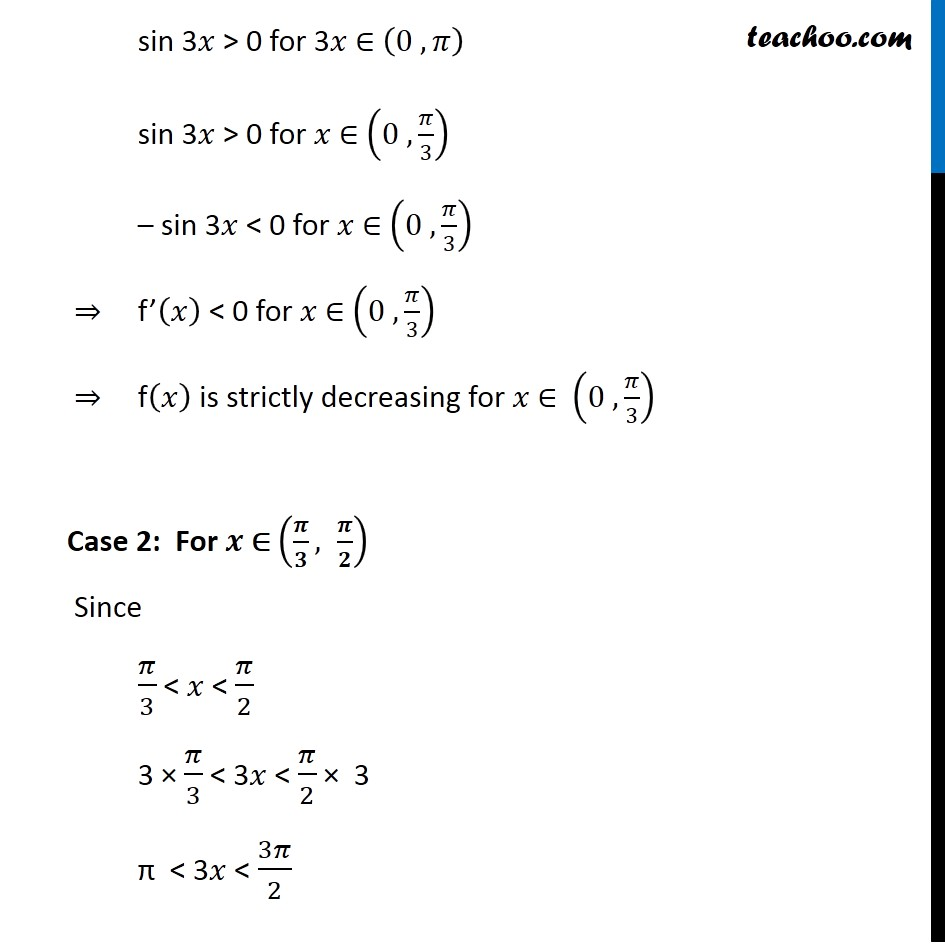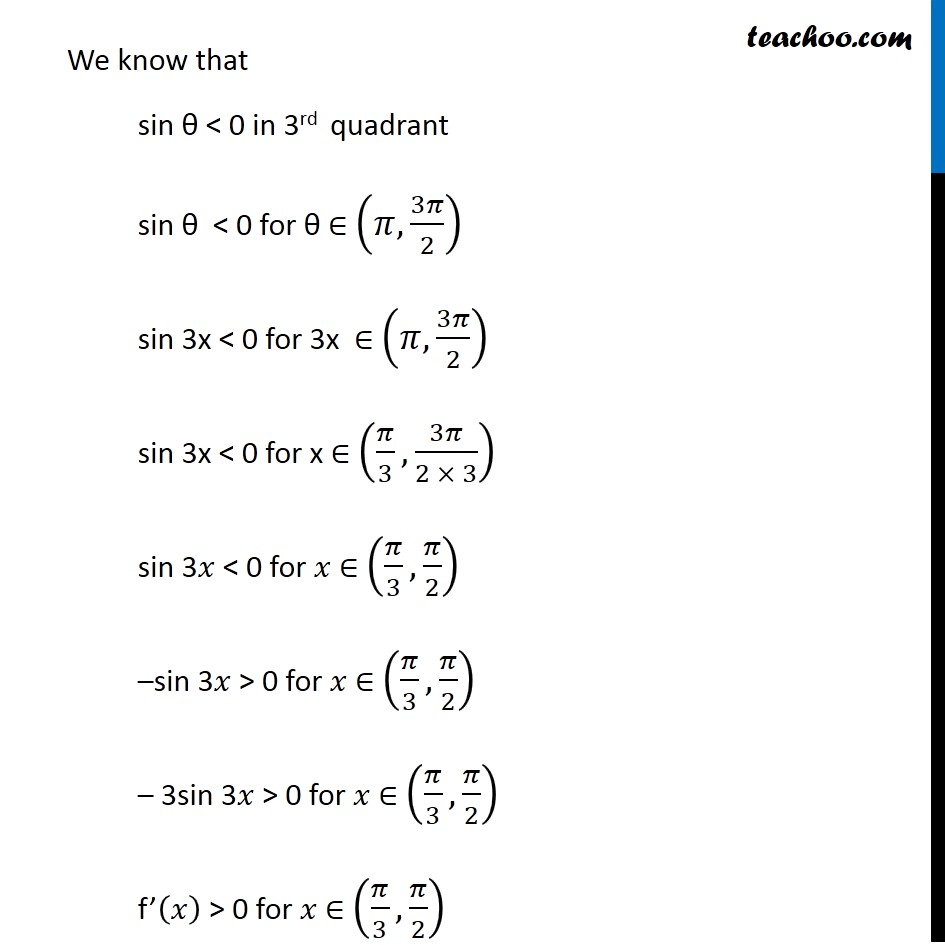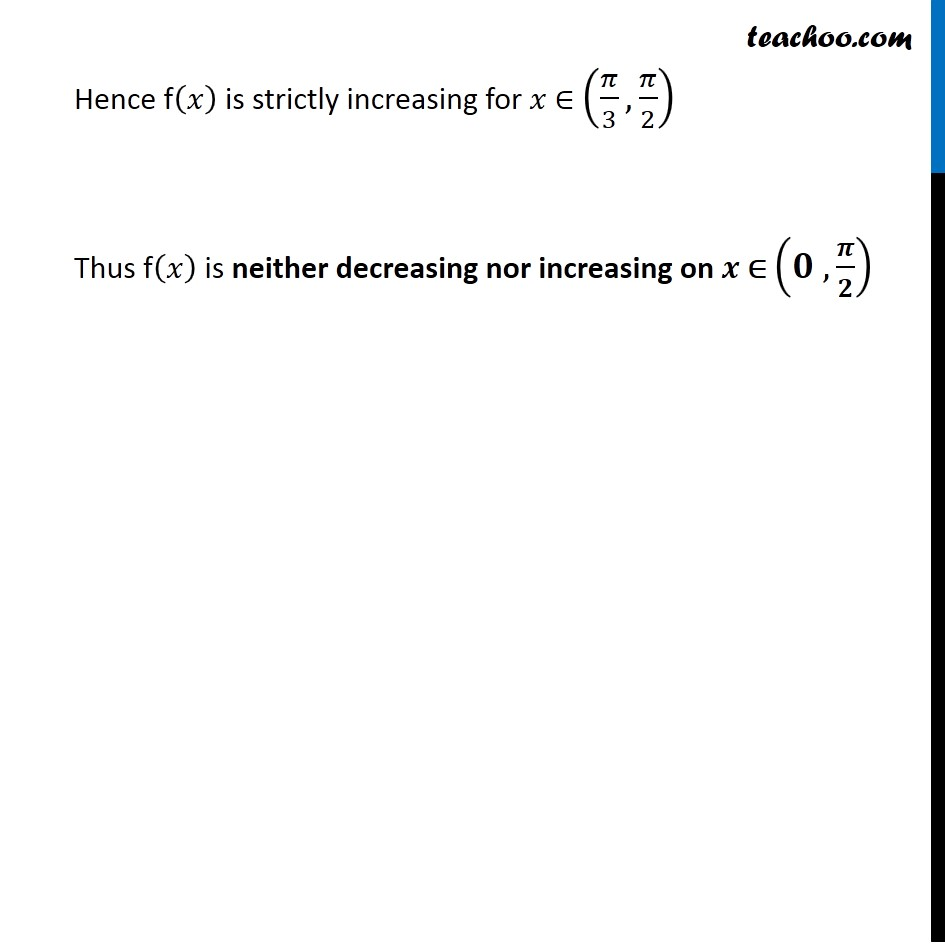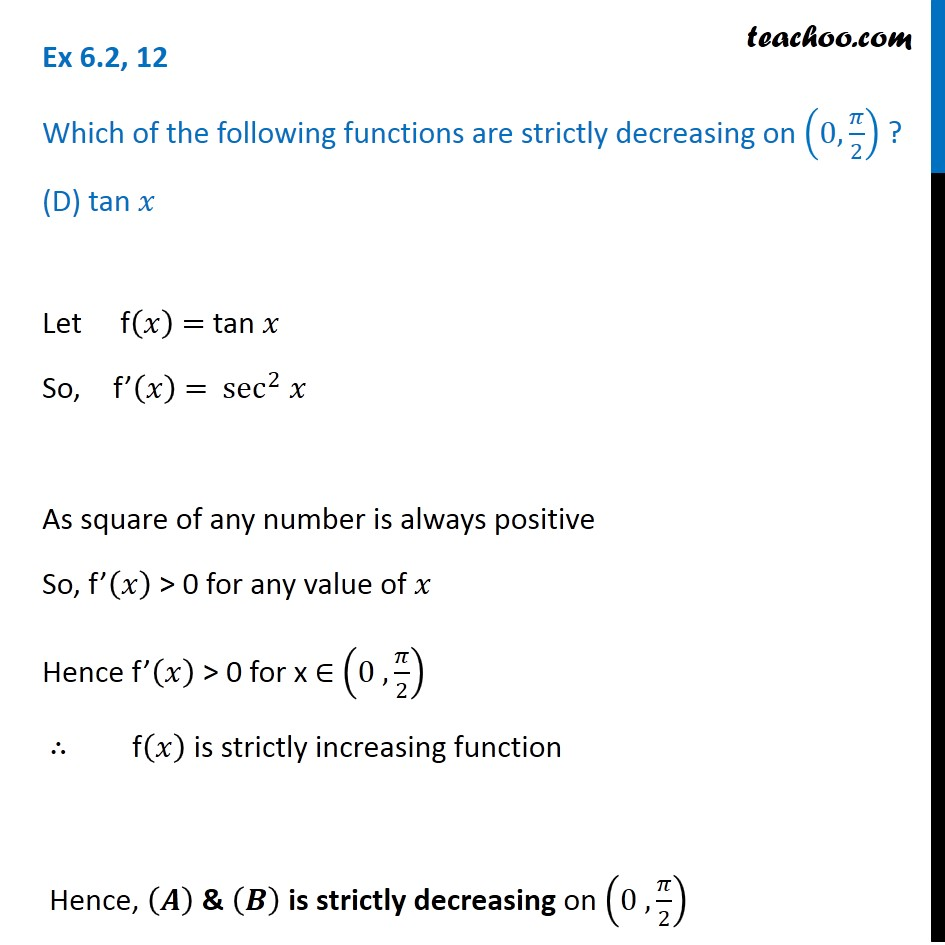1. Chapter 6 Class 12 Application of Derivatives
2. Serial order wise
3. Ex 6.2

Transcript

Ex 6.2,12 Which of the following functions are strictly decreasing on (0 ,𝜋/2)? (A) cos 𝑥 Let f(𝑥) = cos 𝑥 Finding f’(𝑥) f’(𝑥) = – sin 𝑥 Hence, f’(𝑥) < 0 for 𝑥 ∈ (0 , 𝜋/2) ⇒ f(𝑥) is strictly decreasing on (𝟎 , 𝝅/𝟐) Note :- sin 𝑥 > 0 for 𝑥 ∈ (0 , 𝜋/2) So, – sin 𝑥 < for 𝑥 ∈ (0 , 𝜋/2) f’ (𝑥)<0 𝑓𝑜𝑟 𝑥 ∈ 𝜋/2 Ex 6.2,12 Which of the following functions are strictly decreasing on (0,𝜋/2) ? (B) cos 2𝑥 Let f(𝑥) = cos 2𝑥 Finding f’(𝒙) f’(𝑥) = (cos⁡2𝑥 )′ f’(𝑥) = – 2 sin 2𝑥 Putting f’(𝒙) = 0 f’(𝑥) = 0 – 2 sin 2𝑥 = 0 sin 2𝑥 = 0 As 𝑥 ∈ (0 , 𝜋/2) 0 < 𝑥 < 𝜋/2 0 × 2 < 2𝑥 < 𝜋/2 × 2 0 < 2𝑥 < π We know that sin θ > 0 for θ ∈ (0 , 𝜋) sin 2𝑥 > 0 for 2𝑥 ∈ (0 , 𝜋) –sin 2𝑥 < 0 for 2𝑥 ∈ (0 , 𝜋) – 2 sin 2𝑥 < 0 for 𝑥 ∈ (0 , 𝜋/2) ⇒ f’(𝑥) < 0 for 𝑥 ∈ (0 , 𝜋/2) ⇒ f (𝑥) is strictly decreasing for 𝒙 ∈ (𝟎 , 𝝅/𝟐) Ex 6.2,12 Which of the following functions are strictly decreasing on (0,𝜋/2) ? (C) cos 3𝑥 Let f(𝑥) = cos 3𝑥 as 𝑥 ∈ (0 ,𝜋/2) Finding f’(𝒙) f’(𝑥) = (cos⁡3𝑥 )^′ f’(𝑥) = –3 sin 3𝑥 Putting f’(𝒙) = 0 –3 sin 3𝑥 = 0 sin 3𝑥 = 0 As sin θ = 0 if θ = 0 , π , 2π , 3π ∴ 3𝑥 = π , 2π 𝑥 = 𝜋/3 , 2𝜋/3 As 𝑥 ∈ (0 , 𝜋/2) So, 𝑥 = 𝜋/3 Since 𝑥 ∈ (0 , 𝜋/2), so we start number line from (0 , 𝜋/2) The point x = 𝜋/3 divide the interval (0 , 𝜋/2) into 2 disjoint interval. i.e. (0 , 𝜋/3) & (𝜋/3 , 𝜋/2) Checking sign of f’(𝑥) Case 1: For 𝒙 ∈ (𝟎 , 𝝅/𝟑) Now, 0 < 𝑥 < 𝜋/3 3 × 0 < 3𝑥 < 3𝜋/3 0 < 3𝑥 < π So, when 𝑥 ∈ (0 , 𝜋/3) , 3𝑥 ∈ (0 , 𝜋) We know that sinθ > 0 for θ ∈ (0 , 𝜋) sin 3𝑥 > 0 for 3𝑥 ∈ (0 , 𝜋) sin 3𝑥 > 0 for 𝑥 ∈ (0 , 𝜋/3) – sin 3𝑥 < 0 for 𝑥 ∈ (0 ,𝜋/3) ⇒ f’(𝑥) < 0 for 𝑥 ∈ (0 , 𝜋/3) ⇒ f(𝑥) is strictly decreasing for 𝑥 ∈ (0 ,𝜋/3) Case 2: For 𝒙 ∈ (𝝅/𝟑, 𝝅/𝟐) Since 𝜋/3 < 𝑥 < 𝜋/2 3 × 𝜋/3 < 3𝑥 < 𝜋/2 × 3 π < 3𝑥 < 3𝜋/2 We know that sin θ < 0 in 3rd quadrant sin θ < 0 for θ ∈ (𝜋, 3𝜋/2) sin 3x < 0 for 3x ∈ (𝜋, 3𝜋/2) sin 3x < 0 for x ∈ (𝜋/3, 3𝜋/(2 × 3)) sin 3𝑥 < 0 for 𝑥 ∈ (𝜋/3, 𝜋/2) –sin 3𝑥 > 0 for 𝑥 ∈ (𝜋/3, 𝜋/2) – 3sin 3𝑥 > 0 for 𝑥 ∈ (𝜋/3, 𝜋/2) f’(𝑥) > 0 for 𝑥 ∈ (𝜋/3, 𝜋/2) Hence f(𝑥) is strictly increasing for 𝑥 ∈ (𝜋/3, 𝜋/2) Thus f(𝑥) is neither decreasing nor increasing on 𝒙 ∈ (𝟎 , 𝝅/𝟐) Ex 6.2, 12 Which of the following functions are strictly decreasing on (0,𝜋/2) ? (D) tan 𝑥 Let f(𝑥) = tan 𝑥 So, f’(𝑥) = sec^2 𝑥 As square of any number is always positive So, f’(𝑥) > 0 for any value of 𝑥 Hence f’(𝑥) > 0 for x ∈ (0 , 𝜋/2) ∴ f(𝑥) is strictly increasing function Hence, (𝑨) & (𝑩) is strictly decreasing on (0 , 𝜋/2)

Ex 6.2